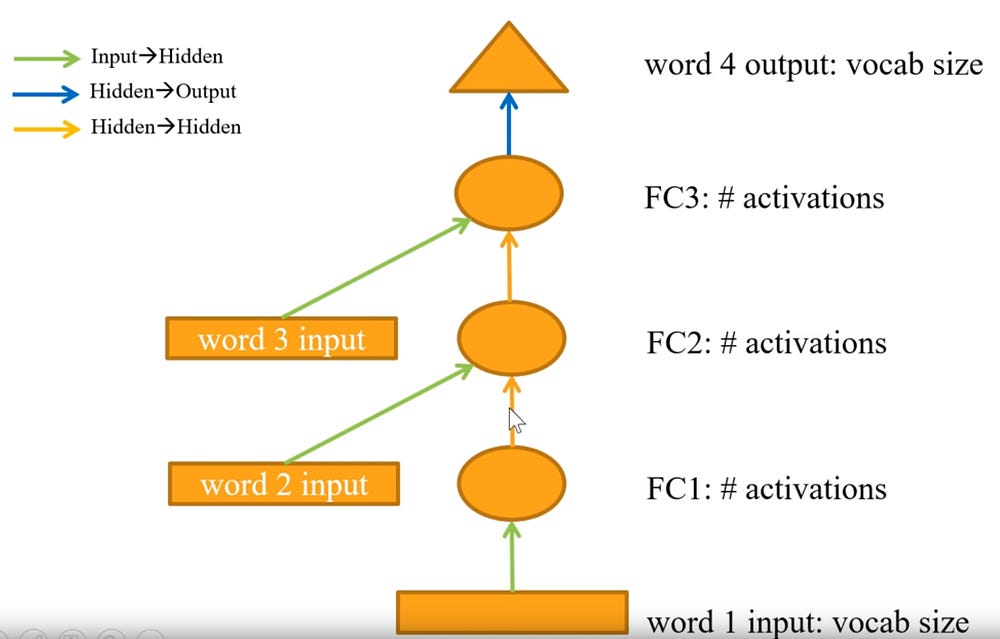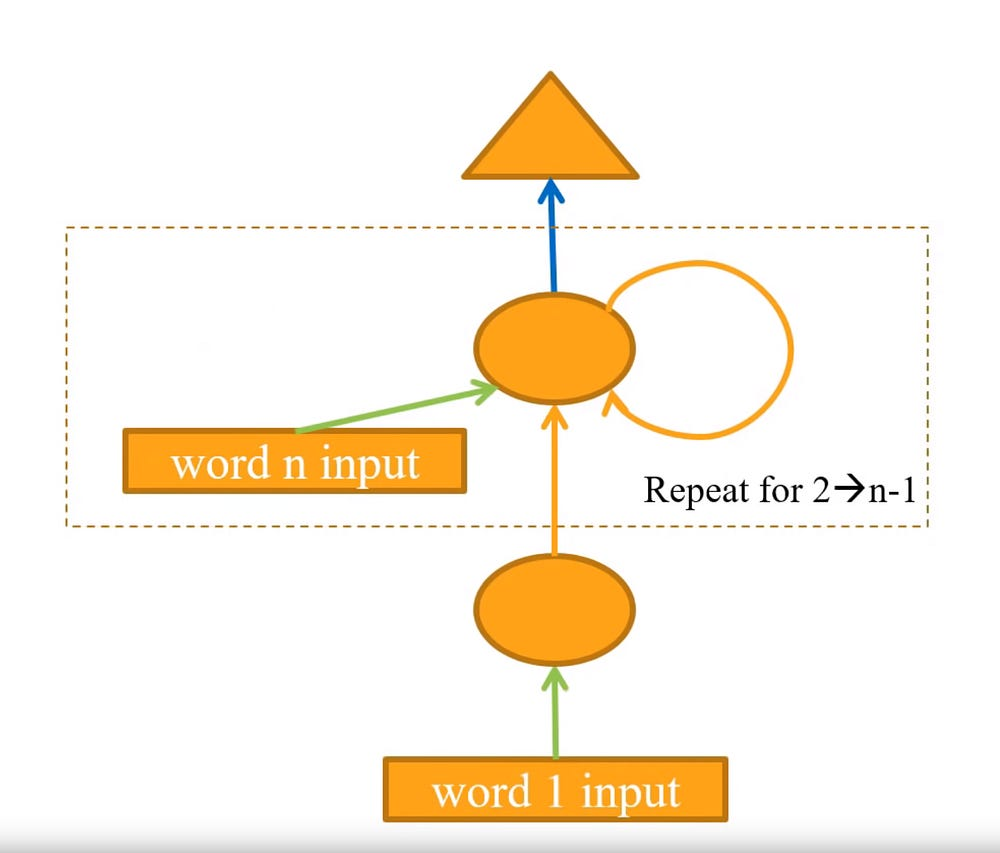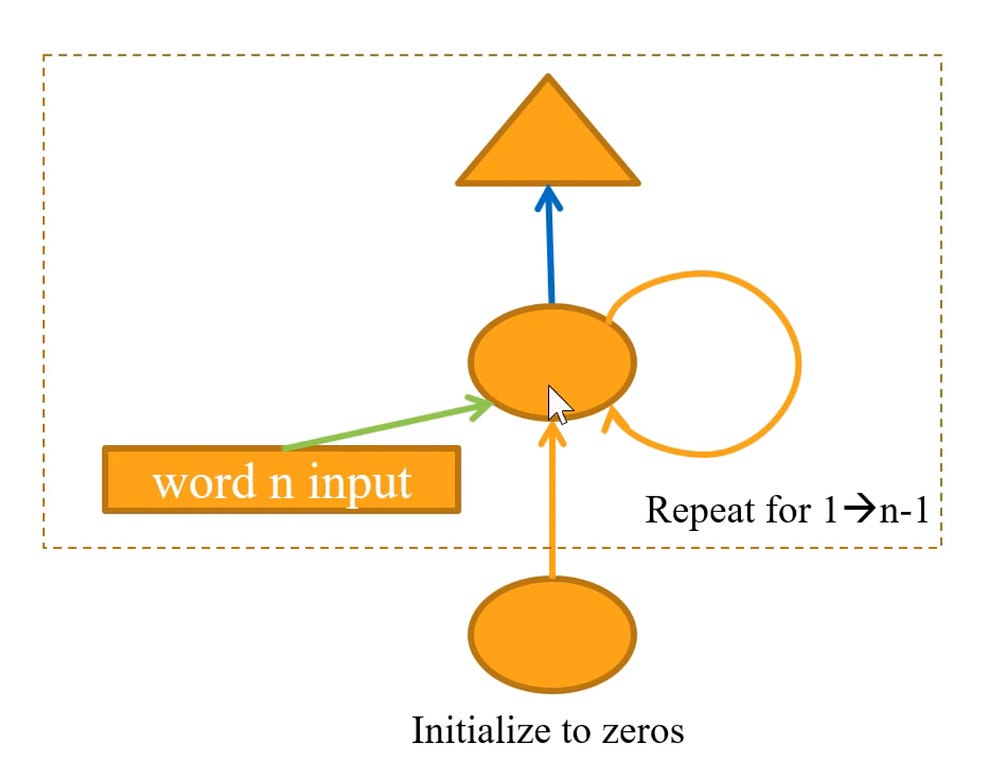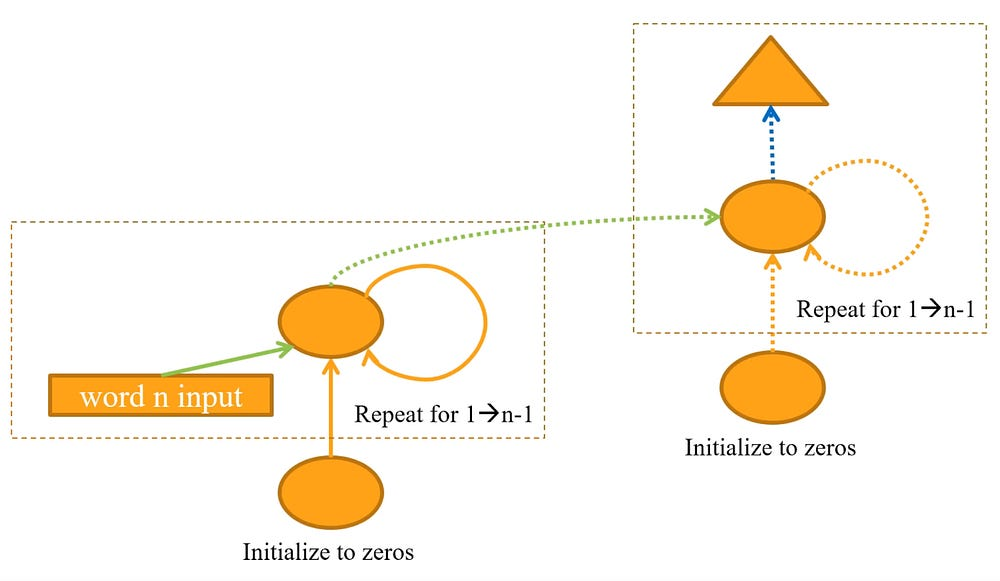Recurrent Neural Networks and Natural Language Processing.

Source: Deep Learning on Medium

Recurrent Neural Networks (RNNs) are a form of machine learning algorithm that are ideal for sequential data such as text, time series, financial data, speech, audio, video among others.

RNNs are ideal for solving problems where the sequence is more important than the individual items themselves.

An RNNs is essentially a fully connected neural network that contains a refactoring of some of its layers into a loop. That loop is typically an iteration over the addition or concatenation of two inputs, a matrix multiplication and a non-linear function.

Among the text usages, the following tasks are among those RNNs perform well at:

• Sequence labelling
• Natural Language Processing (NLP) text classification
• Natural Language Processing (NLP) text generation

Other tasks that RNNs are effective at solving are time series predictions or other sequence predictions that aren’t image or tabular based.

There has been several highlighted and controversial reports in the media over the advances in text generation, in particular OpenAI’s GPT-2 algorithm. In many cases the generated text is often indistinguishable from text written by humans.

I found learning how RNNs function and how to construct them and their varients has been among the most difficult topics I have had to learn. I would like to thank the Fastai team and Jeremy Howard for their courses explaining the concepts in amore understandable order, which I’ve followed in this article’s explanation.

RNNs effectively have an internal memory that allows the previous inputs to affect the subsequent predictions. It’s much easier to predict the next word in a sentence with more accuracy, if you know what the previous words were.

Often with tasks well suited to RNNs, the sequence of the items is as or more important than the previous item in the sequence.

As I’m typing the draft for this on my smart phone, the next word suggested by my phone’s keyboard will be predicted by an RNN. For example, the swift key keyboard software uses RNNs to predict what you are typing.

Natural Language Processing

Natural Language Processing (NLP) is a sub-field of computer science and artificial intelligence, dealing with processing and generating natural language data. Although there is still research that is outside of the machine learning, most NLP is now based on language models produced by machine learning.

NLP is a good use case for RNNs and is used in the article to explain how RNNs can be constructed.

Language models

The aim for a language model is to minimise how confused the model is having seen a given sequence of text.

It is only necessary to train one language model per domain, as the language model encoder can be used for different purposes such as text generation and multiple different classifiers within that domain.

As the longest part of training is usually creating the language model encoder, reusing the encoder can save significant training time.

Comparing an RNN to a fully connected neural network

If we take a sequence of three words of text and a network that predicts the fourth word.

The network has three hidden layers, each of which are an affine function (for example a matrix dot product multiplication), followed by a non-linear function then the last hidden layer is followed by an output from the last layer activation function.

The input vectors representing each word in the sequence are lookups in a word embedding matrix, based on a one hot encoded vector representing the word in the vocabulary. Note that all inputted words use the same word embedding. In this context a word is actually a token that could represent a word or a punctuation mark.

The output will be a one hot encoded vector representing the predicted fourth word in the sequence.

The first hidden layer takes a vector representing the first word in the sequence as an input and the output activations serve as one of the inputs into the second hidden layer.

The second hidden layer takes the input from the activations of the first hidden layer and also an input of the second word represented as a vector. These two inputs could be either added or concatenated together.

The third hidden layer follows the same structure as the second hidden layer, taking the activation from the second hidden layer combined with the vector representing the third word in the sequence. Again, these inputs are added or concatenated together.

The output from the last hidden layer goes through an activation function that produces an output representing a word from the vocabulary, as a one hot encoded vector.

This second and third hidden layer could both use the same weight matrix, opening the opportunity of refactoring this into a loop to become recurrent.A fully connected network for text generation/prediction. Source: Fastai deep learning course V3 by Jeremy Howard.

Vocabulary

The vocabulary is a vector of numbers, called tokens where each token represents one of the unique words or punctuation symbols in our corpus.

Usually words that don’t occur at least twice in the texts making up the corpus usually aren’t included, otherwise the vocabulary would be too large. I wonder if this could be used as a factor for detecting generating text, looking for the presence of words not common in the given domain.

Word embedding

A word embedding is a matrix of weights, with a row for each word/token in the vocabulary

Matrix dot product multiplication with a one hot encoded vector outputs a row of the matrix representing activations from that word. It is essentially a row lookup in the matrix and is computationally more efficient to do that, this is called an embedding lookup.

Using the vector from the word embedding helps prevent the resulting activations being very sparse. As if the input was the one hot encoded vector, which is all zeros apart from one element, the majority of the activations would also be zero. This would then be difficult to train.

Refactored with a loop, an RNN

For the network to be recurrent, a loop needs to be factored into the network’s model. It makes sense to use the same embedded weight matrix for every word input. This means we can replace the second and third layers with iterations within a loop.

Each iteration of the loop takes an input of a vector representing the next word in the sequence with the output activations from the last iteration. These inputs are added or concatenated together.

The output from the last iteration is a representation of the next word in the sentence being put through the last layer activation function which converts it to a one hot encoded vector representing a word in the vocabulary.A basic RNN. Source: Fastai deep learning course V3 by Jeremy Howard.

This allows the network to predict a word at the end of a sequence of any arbitrary length.

Retaining the output through out the loop, an improved RNN

Once at the end of the sequence of words, the predicted output of the next word could be stored, appended to an array, to be used as additional information in the next iteration. Each iteration then has access to the previous predictions.

For a given number of inputs there are the same number of outputs created.An improved RNN retaining its output. Source: Fastai deep learning course V3 by Jeremy Howard.

In theory the sequence of predicted text could be infinite in length, with a predicted word following the last predicted word in the loop.

Retaining the history, a further improved RNN

With each new batch the history of the previous batch’s sequence, the state, is often lost. Assuming the sentences are related, this may lose important insights.

To aid the prediction when we start each batch, it is helpful to know the history of the last batch rather than reset it. This retains the state and hence the context, this results in an understanding of the words that is a better approximation.

Note with some datasets such as one-billion-words each sentence isn’t related to the previous one, in this case this may not help as there is no context between sentences.

Backpropagation through time

Back propagation through time (BPTT) is the sequence length used during training. If we were trying to train on sequences of 50 words, the BPTT would be 50.

Usually the document is split into 64 equal sections. In this case the BPTT is the document length in words divided by 64. If the document length in words is 3200 then that divided by 64 gives a BPTT of 50.

It’s beneficial to slightly randomise the BPTT value for each sequence to help improve the model.

Layered RNNs

To get more layers of computation to be able to solve or approximate more complex tasks, the output of the RNN could be fed into another RNN, or any number of layers of RNNs. The next section explains how this can be done.

Extending RNNs to avoid the vanishing gradient

As the number of layers of RNNs increases the loss landscape and can become impossible to train, this is the vanishing gradient problem. To solve this problem a Gated Recurrent Unit (GRU) or a Long Term Short Term Memory (LSTM) network can be used.

LSTMs and GRUs take the current input and previous hidden state, then compute the next hidden state.

As part of this computation, the sigmoid function squashes the values of these vectors between 0 and 1, and by multiplying them elementwise with another vector you define how much of that other vector you want to “let through”

Long Term Short Term Memory (LSTM)

An RNN has short term memory. When used in combination with Long Short Term Memory (LSTM) Gates, the network can have long term memory.

Instead of the recurring section of an RNN, an LTSM is a small neural network consisting of four neural network layers. These are the recurring layer from the RNN with three networks acting as gates.

An LSTM also has a cell state as well, along side the hidden state. This cell state is the long term memory. Rather than just returning the hidden state at each iteration, a tuple of hidden states are returned comprised of the cell state and hidden state.

Long Short Term Memory (LSTM) has three gates.

1. An Input gate, this controls the information input at each time step.
2. An Output gate, this controls how much information is outputted to the next cell or upward layer
3. A Forget gate, this controls how much data to lose at each time step.

Gated recurrent unit (GRU)

A gated recurrent unit is sometimes referred to as a gated recurrent network.

At the output of each iteration there is a small neural network with three neural networks layers implemented, consisting of the recurring layer from the RNN, a reset gate and an update gate. The update gate acts as a forget and input gate. The coupling of these two gates performs a similar function as the three gates forget, input and output in an LSTM.

Compared to an LSTM, a GRU has a merged cell state and hidden state, whereas in an LSTM these are separate.

Reset gate

The reset gate takes the input activations from last layer, these are multiplied by a reset factor between 0 and 1. The reset factor is calculated by a neural network with no hidden layer (like a logistic regression), this performs a dot product matrix multiplication between a weight matrix and the addition/concatenation of the previous hidden state and our new input. This is then all put through the sigmoid function e^x / (1 + e^x).

This can learn to do different things in different situations, for example to forget more information if there’s a full stop token.

Update gate

The update gate controls how much of the new input to take and how much of the hidden state to take. This is a linear interpolation. This is 1 — Z multiplied by the previous hidden state plus Z multiplied by the new hidden state. This controls to what degree we keep information from the previous states and to what degree we use information from the new state.

The update gate is often represented as a switch in diagrams, although the gate can be in any position to create a linear interpolation between the two hidden states.A RNN with a GRU. Source: Fastai deep learning course V3 by Jeremy Howard.

Which is better, a GRU or an LSTM

This depends entirely on the task in question, it is often worth trying both to see which can perform better.

Text classification

In text classification the prediction of the network is to classify which group or groups the text belongs to. A common use is classifying if the sentiment of a piece of text is positive or negative.

If an RNN is trained to predict text from a corpus within a given domain as in the RNN explanation earlier in this article, it is close to ideal to be re-purposed for text classification within that domain. The generation ‘head’ of the network is removed leaving the ‘backbone’ of the network. The weights within the backbone can then be frozen. A new classification head can then be attached to the backbone and trained to predict the required classifications.

It can be a very effective method to speed up training to gradually unfreeze the weights within the layers. Starting with the weights of the last two layers, then the weights of the last three layers, and finally all unfreeze all of the layers’ weights.

Transfer learning and the Wikitext 103 data set

The wikitext 103 dataset contains over 103 million tokens from good or featured tokens from Wikipedia.

A pretrained model is available trained on the wikitext 103 dataset, this can be used for transfer learning for almost any language processing task. It is much closer to any written text than any random initialisation ever could be. It’s one of those contradictions to the no free lunch theory. Most of the time it gets the algorithm close to the solution before you’ve even started training.

Fastai courses

I would like to thank the Fastai team whose courses have helped cement my deep learning and RNN knowledge providing an excellent base for further learning and understanding.